Courses

# Test: Probability And Expected Value By Mathematical Expectation- 8

## 40 Questions MCQ Test Business Mathematics and Logical Reasoning & Statistics | Test: Probability And Expected Value By Mathematical Expectation- 8

Description
This mock test of Test: Probability And Expected Value By Mathematical Expectation- 8 for CA Foundation helps you for every CA Foundation entrance exam. This contains 40 Multiple Choice Questions for CA Foundation Test: Probability And Expected Value By Mathematical Expectation- 8 (mcq) to study with solutions a complete question bank. The solved questions answers in this Test: Probability And Expected Value By Mathematical Expectation- 8 quiz give you a good mix of easy questions and tough questions. CA Foundation students definitely take this Test: Probability And Expected Value By Mathematical Expectation- 8 exercise for a better result in the exam. You can find other Test: Probability And Expected Value By Mathematical Expectation- 8 extra questions, long questions & short questions for CA Foundation on EduRev as well by searching above.
QUESTION: 1

Solution:
QUESTION: 2

Solution:
QUESTION: 3

### Consider two events A and B not mutually exclusive, such that P(A) = 1/4, P(B) =2/5, P(A∪B) = 1/2, then P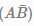is

Solution:
QUESTION: 4

The probability of Girl getting scholarship is 0.6 and the same probability for Boy is 0.8. Find the probability that at least one of the categories getiing scholarship.

Solution:
QUESTION: 5

In a pack of playing cards with two jokers probability of getting king of spade is

Solution:
QUESTION: 6

The odds in favour of A solving a problem is 5:7 and Odds against B solving the same problem is 9:6. What is the probability that if both of them try, the problem will be solved?

Solution:
QUESTION: 7

If x be the sum of two numbers obtained when two die are thrown simultaneously then P(x ≥ 7) is

Solution:
QUESTION: 8

E(13x+9)=___________.

Solution:
QUESTION: 9

The probability of winning of a person is 6/11 and at a result he gets Rs.77/-. The expectation of this person is

Solution:
QUESTION: 10

E(XY) is also known as:

Solution:
QUESTION: 11

If P(A/B) = P

Solution:
QUESTION: 12

If P(A∪B) = P(A), Find P(A∩B).

Solution:
QUESTION: 13

Consider Urn I :2 white balls, 3 black balls
Urn II : white balls, 6 black balls
One ball is randomly transferred from first to second Urn, then one ball is drawn from II Urn. The probability that drawn ball is white is

Solution:
QUESTION: 14

A bag contains 3 white and 5 black balls and second bag contains 4 white and 2 black balls. If one balls is taken from each bag, the probability that both the balls are white is___________

Solution:
QUESTION: 15

If A and B are two independent events and P(AUB) =2/5; P(B) = 1/3. Find P(A).

Solution:
QUESTION: 16

In a throw of dice what is the probability of getting number greater than 5

Solution:

Number greater than 5 is 6, so only 1 number
Total cases of dice = [1,2,3,4,5,6]
So probability = 1/6

QUESTION: 17

If 10 men, among whom are A and B, stand in a row, what is the probability that there will be exactly 3 men between A and B?

Solution:
QUESTION: 18

The probability of an event can assume any value between:

Solution:
QUESTION: 19

If P(A∩B) = P(A) x P(B), then the events are:

Solution:
QUESTION: 20

A random variable X has the following probability distribution: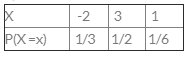Find E (X2) and E(2X+5).

Solution:
QUESTION: 21

A bag contains 12 balls of which 3 are red 5 balls are drawn at random. Find the probability that in 5 balls 3 are red.

Solution:
QUESTION: 22

The probability that a trainee will remain with a company is 0.8. The probability that an employee earns more than Rs. 20,000 per month is 0.4. The probability that an employee, who was a trainee and remained with the company or who earns more than Rs. 20,000 per month is 0.9. What is the probability that an employee earns more than Rs. 20.000 per month given that he is a trainee, who stayed with the company?

Solution:
QUESTION: 23

Daily demand for calculators is having the following probability distribution: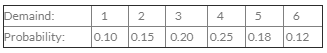Determine the variance of the demand:

Solution:
QUESTION: 24

P(A) = 2/3; P(B) = 3/5; P(A∪ B) = 5/6. Find P (B/A)

Solution:
QUESTION: 25

The odds are 9:5 against a person who is 50 years living till he is 70 and 8:6 against a person who is 60 living till he is 80. Find the probability that at least one of them will be alive after 20 years:

Solution:
QUESTION: 26

An urn contains 6 white and 4 black balls. 3 balls are drawn without replacement. What is the expected number of black balls that will be obtained?

Solution:
QUESTION: 27

A random variable X has the following probability distribution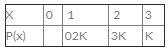Then, P(x<3) would be:

Solution:
QUESTION: 28

The limiting relative frequency of probability is:

Solution:
QUESTION: 29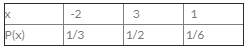then find E(2x+5)

Solution:
QUESTION: 30

The theory of compound probability states that for any two events A and B:

Solution:
QUESTION: 31

In a non-leap year, the probability of getting 53 Sundays or 53 Tuesdays or 53 Thursday is

Solution:
QUESTION: 32

Suppose E and F are two events of a random experiment. If the probability of occurrence of E is 1/5 and the probability of occurrence of F given E is 1/10, then the probability of non-occurrence of at least one of the events E and F is:

Solution:
QUESTION: 33

Among the examinees in an examination 30%, 35% and 45% failed in Statistic, in Mathematics and in at least one of the subjects respectively. An examinee is selected at random. Find the probability that he failed in Mathematics only:

Solution:
QUESTION: 34

From a pack of cards, two are drawn, the first being replaced before the second is drawn. The change that the first is a  second is drawn.

Solution:
QUESTION: 35

Amitabh plays a game of tossing a dice. If the number less than 3 appears, he is getting Rs. a, otherwise he has to pay Rs. 10. If the game is fair, find a:

Solution:
QUESTION: 36

A card is drawn from a well shuffled pack of 52 cards. Let E1 “a king or a queen is drawn” & E2: “a queen or a jack is drawn”, then:

Solution:
QUESTION: 37

The probability that there is at least one error in an account statement prepared by A is 0.3 and for B and C, they are 0.4 and 0.45 respectively. A, B and C prepared 20, 10 and 40 statements respectively. The expected number of correct statement respectively. The expected number of correct statements in all is:

Solution:
QUESTION: 38

A box contains 12 electric lamps of which 5 are defectives. A man selects three lamps at random. What is the expected number of defective lamps in his selection?

Solution:
QUESTION: 39

Three identical dice are rolled. The probability that the same number will appear on each of them is:

Solution:
QUESTION: 40

An urn contains 9 balls two of which are red, three blue and four black. Three balls are drawn at random. The probability that they are of same colour is :

Solution: